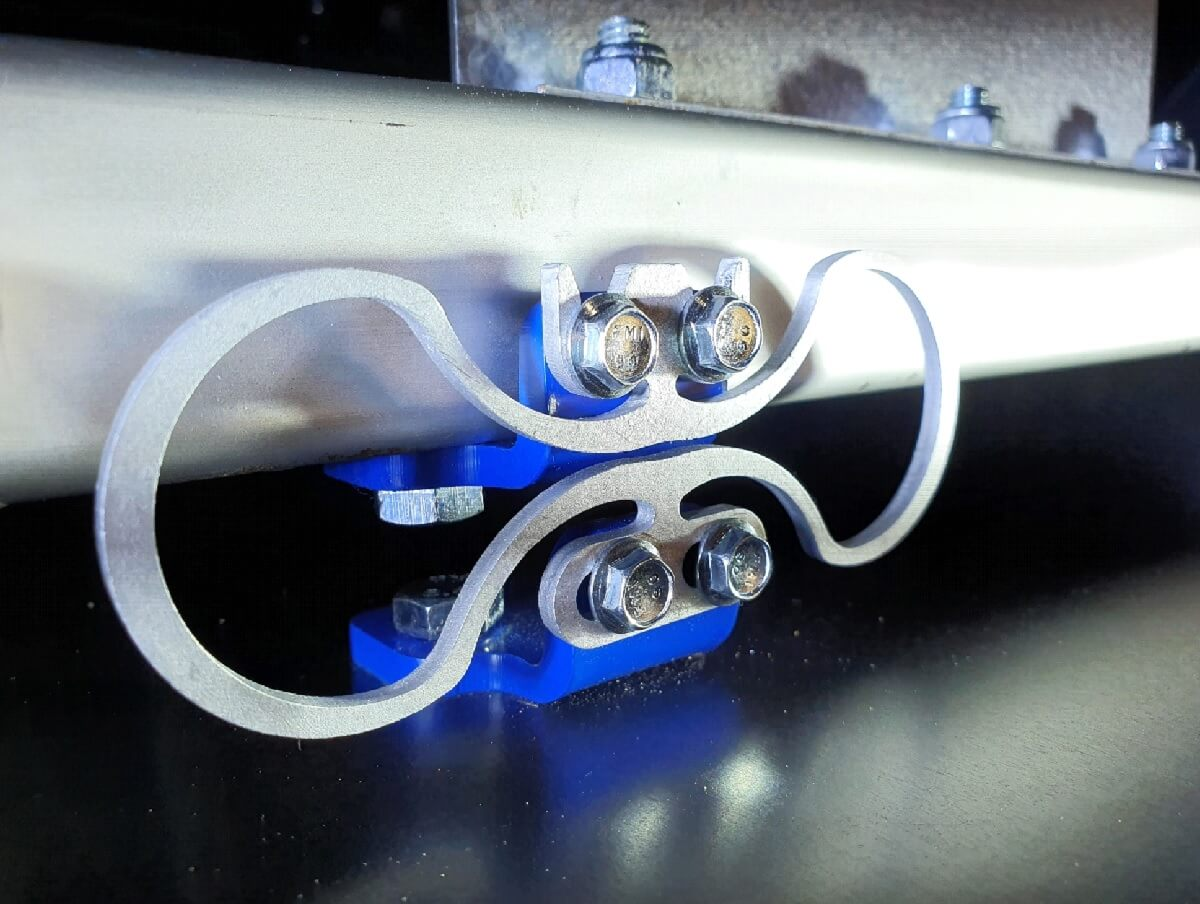Test

24.09.2020

### Fan Introduction

The test case fan is equipped with a 2.2 kW electric motor, which is controlled via variable-frequency drive. The motor nominal speed is 1440 rpm. The fan is used in air handling units and typically equipped with rubber elements. In this article InfiniSpring®-springs are dimensioned below the fan.

#### Fan weight

The fan weight was first measured to determine spring selection. Measurement results were taken below the existing rubber elements and measurement results are shown in Figure 1.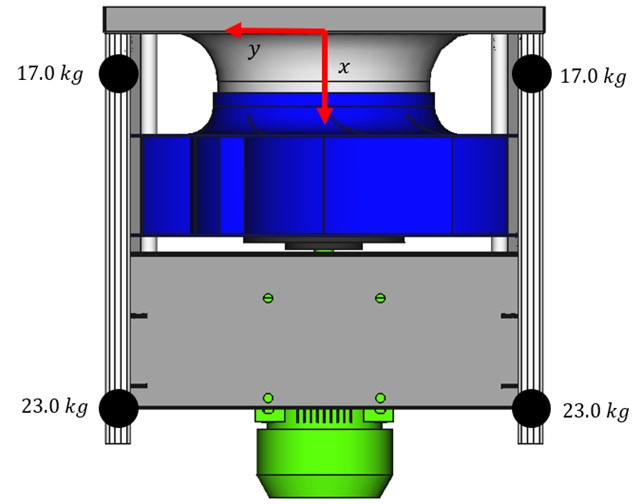Figure 1. Weight measurement results for the fan.

#### Spring selection

The fan originally had four springs and four InfiniSpring®-springs were chosen to be used in the fan. Spring selection can be made with following steps:

• Calculating total mass: 17.0 kg + 17.0 kg + 23.0 kg + 23.0 kg = 80.0 kg
• Deciding required number of springs: 4
• Calculation load for one individual spring: 80 kg / 4 = 20 kg
• Choosing optimal spring based on the individual spring load: 3HS20 spring with nominal load range 15-20 kg according to Table 1 below (table is taken from the datasheet found on the Product page)

####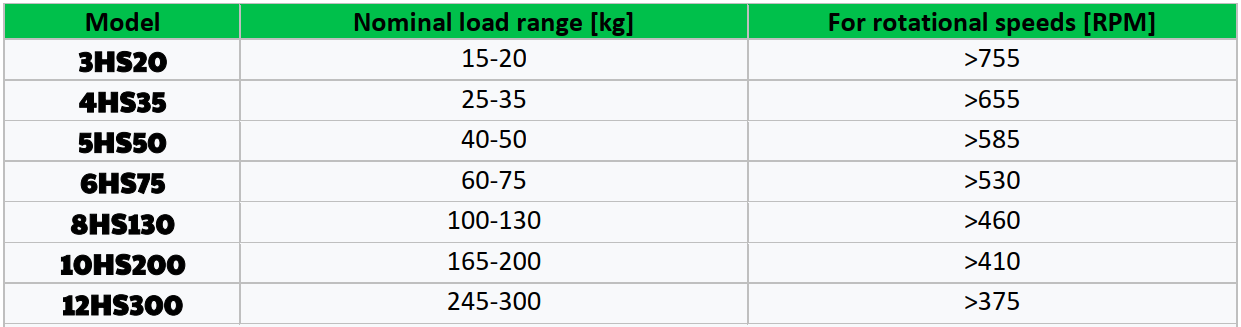Table 1. Vertical nominal loads of a single spring from the datasheet.

#### Spring locations

Final step is to dimension springs symmetrically around the center of gravity in the fan axial direction. Fan motor axial direction is referred as X-direction in Figure 2. The zero-point location is also shown in Figure 2.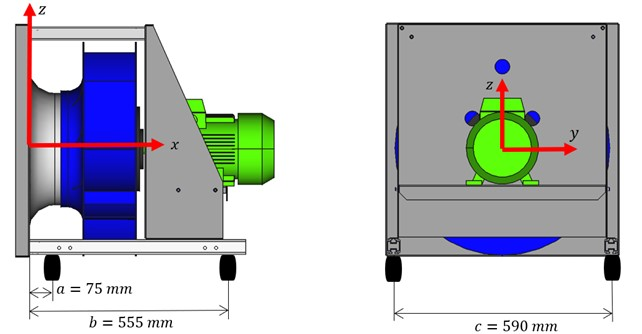Figure 2. Fan equipped with original rubber elements.

First the centre of mass x-coordinate is calculated. After this the location for the motor end spring is chosen. In this case 65 mm from the end of the 584 mm long attachment aluminum bar is used, Figure 3. Spring locations from the zero-point location are then calculated as follows.

#### Calculation steps

• Calculating centre of mass x-coordinate: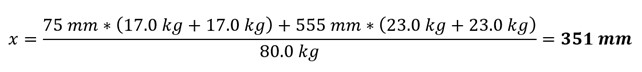• Deciding motor end spring location: 65 mm
• Calculating spring locations: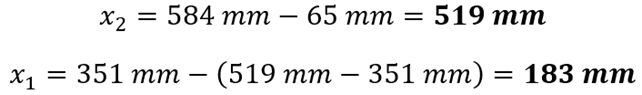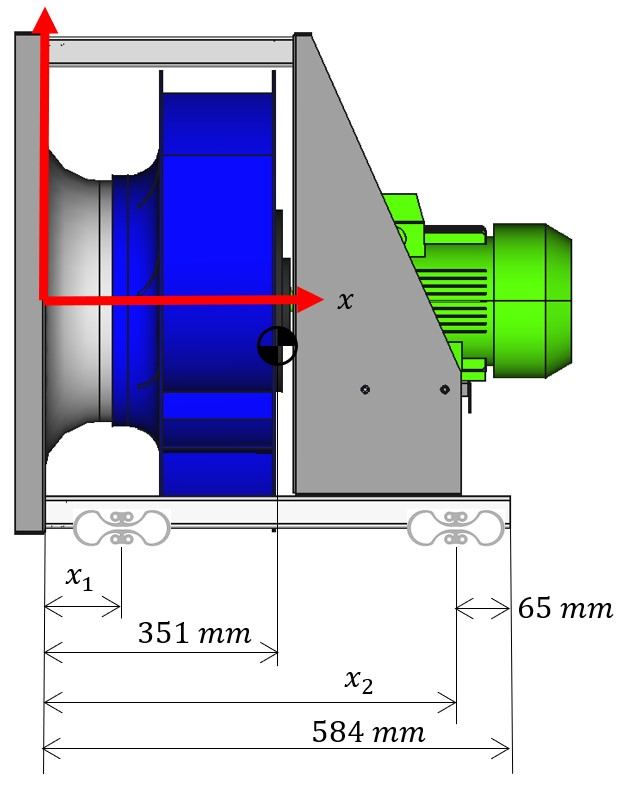Figure 3. Spring locations

#### Fan with new springs

Fan with 3HS20 InfiniSpring® is shown below in Figure 4. Individual InfiniSpring® is shown in Figure 5. Optimal springs were selected for the fan and the fan is ready for operation. Safe operational speed ranges from 755 rpm upwards. Minimum operational speeds can be found from the and also shown in Table 1 above. Please see also a video below introducing the fan and InfiniSpring®-springs.Figure 4. Fan equipped with 3HS20 InfiniSpring®